Disclaimer: This is an example of a student written essay.

Any opinions, findings, conclusions or recommendations expressed in this material are those of the authors and do not necessarily reflect the views of UKEssays.com.

# Simplified Soft Decision Decoding of LDPC codes

 ✅ Paper Type: Free Essay ✅ Subject: Communications ✅ Wordcount: 1867 words ✅ Published: 26th Jul 2018

• MARUTHI L N
• K S GURUMURTHY

### Abstract

LDPC codes gained importance since its re-discovery by Mackay and Neil based on Tanner Graph. This paper presents the implementation of forward error correction soft-input, soft-output decoding process that efficiently decodes the received set of data under low signal-to-noise ratios due to which the errors are reduced and hence transmission time is greatly reduced. The implemented algorithm is less complex and does not require knowledge of signal-to-noise-ratio of the received data-path.

## INTRODUCTION

Error correction coding techniques came into existence after existence of channel capacity theorem by Shannon, the father of information theory in 1948 on reliable communication over noisy transmission channels. Thereafter, many coding techniques were developed for efficient coding techniques, like Hamming, Golay and many other techniques were developed. Though Gallagher in 1962 developed LDPC codes, the method employed was not optimal. Hence it was not reviewed for last 3 decades, until 1992, when a paper “Near Shannon limit error correcting coding and decoding: turbo codes” presented by Berrou, Glavieux and Thitimajshima changed the trend followed by researchers for the past 5 decades. And now, we are aiming to develop such codes through another strategy. The coding gain provided by this method is much higher when compared to other coding systems. The LDPC codes are becoming more popular because of their reduced power for transmission and less complex logic to achieve low BER’s which is very essential for reliable transmission over noisy channels.

## LDPC CODES

Low Density Parity Check (LDPC) codes are a powerful class of forward error correction codes developed by Gallagher in 1962, practically implemented by MacKay in 1995. Low Density refers to less number of 1’s in the Parity Check Matrix (H) of Block Codes.

Block codes are a type of Error Correction Codes which uses a Generator Matrix(G) to produces a Code-word of length n for message(D) of length k, where n-k is the redundancy bits added to make a Code-word of length n, higher the redundancy higher the error correction capability and higher the Bandwidth. Hence, there is a trade-off between error correction capability and bandwidth.

C=D.G

The size of the Matrix G is (n, n-k) and the size of the Matrix H is (n-k, n).

Usually redundancy is defined by the term “code rate” which is given by

R=k/n

This varies from ½ to 1/6.

The Encoder produces a Code-word which satisfy the condition

C.HT=0

If R is the received data from the Transmitter then the acknowledgement of the correct data received is given by the Syndrome(S).

S=R.HT

The value of S obtained by the above calculation determines whether the received data is free from error or not, i.e. if S=0 then the received data is free from error, otherwise the received data has an error.

Since this property is very useful to reduce the calculation time if we receive the data correctly as soon as we receive data. The probability that the received data needs to be retransmitted which in the range of 10-6 to 10-8.

Encoding the data as required is an easy process, but decoding the data effectively and efficiently is a very hard process.

The proposed algorithm uses the basic concept of block codes, i.e.

R=C+E

Where R=noise corrupted vector

C=Code vector transmitted over noisy channel

E=Error vector.

The receiver does not know C and E; its function is to decode C from R, and the message D from C.

## PROPOSED ALGORITHM

The base for LDPC decoding algorithm proposed is as described by MacKay and Neil in 1997.

Following are the steps that briefly describe the algorithm

STEP 1: INITIALIZATION

Let rj be the received vector, i.e. the data received from the Gaussian channel

We calculate the components of the vectors d02( j), and d12( j) as:

d02( j)= (rj+1)2

d12( j)= (rj-1)2 j = 1, 2, . . . , n (1)

These first soft estimates of the code symbols are used to initialise the algorithm by setting the following coefficients q0ij and q1ij at each symbol node

q0ij= d02( j)

q1ij= d12( j) j = 1, 2, . . . , n,

i = 1, 2. . . , . n-k, (2)

To eliminate the effect of zero in the calculation the expressions for the calculations can be reduced as shown below

ln(em+en) = max(m,n) + ln(1+ e-|m-n|)

log2(2m+2n) = max(m,n) + ln(1+ 2-|m-n|)(3)

The tables required for calculations are computed using the above formula. The complexity is greatly reduced and the reliability of the transmission of signal is increased.

STEP 2: HORIZONTAL STEP

aij= f+(q0ij , q1 ij )

bij= −f−(q0ij , q1ij)

if (−q 0ij ) ≥ (−q1ij )

sij= 0 else

sij= 1

cij=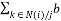ik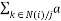ik

if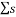ik is even

r0n,ij= −f+(0, cij )

r1n,ij= f−(0, cij )

ifik is odd

r0n,ij= f−(0, cij )

r1n,ij= −f+(0, cij )

STEP 3: VERTICAL STEP

q0ij= d02 (j) +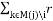0n,ij

q1ij= d12 (j) +1n,ij

STEP 4: DECISION

rm0ij=r0n,ij+ q0ij

rm1ij=r1n,ij+ q1ij

if rm02(j)< rm12(j) then

c(j) = 1

else

c(j) = 0

The LUT entries used in the proposed algorithm for equation (3) and the exact value obtained will vary as shown in the figure.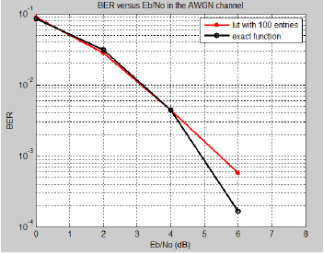Comparison of BER performance of (8 * 12) LDPC SSD decoder (10 iterations)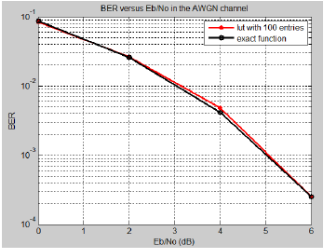Comparison of BER performance of (8 * 12) LDPC SSD decoder (50 iterations)

As explained above, for higher precision the number of iterations is increased to obtain the same performance of the exact equation.

The obtained results approach towards the complex algorithm developed by Gallagher with simple iterative approach and provides a high coding gain compared to uncoded signal. And it provides higher performance at larger iterations. This algorithm provides even higher performance for large length codes.

## CONCLUSION

In this paper we have described Low Density Parity Check (LDPC) codes and decoding of these codes using low complexity algorithms. LDPC codes are used now-a-days in communication systems that take advantage of parallelism, good error correction and high through put. This led to the new algorithm which could decode the errors and yet give similar BER performance as the complex algorithms without the knowledge of the channel noise parameters like variance. This new algorithm is based on repeated use of an antilog-sum operation, and has been simulated on the Tanner graph representation of several LDPC codes and this algorithm can be regarded as a generalized form of belief propagation, where the belief propagated is Euclidean distance estimate rather than a probability estimate. The advantages of the new algorithm are that the performance is as good as the complex algorithm provided the value of base of the logarithm is used properly; that knowledge of noise is not required and that in the simplified form the algorithm needs only additions/subtractions, comparisons and two look-up tables avoiding the use of quotients and products operations that are of high complexity in practical implementations especially using FPGA technology.

## REFERENCES

1] R.G. Gallager, “Low Density Parity Check Codes”, IRE Trans. Information Theory, IT-8, 21-28 (1962).

2] D.J.C. Mackay and R.M. Neal, “Near Shannon limit performance of low density parity check codes,” Electronics Letters, vol. 33, pp 457-458 (1997).

3] L. Arnone, C. Gayaso, C. Gonzalez and J. Castineira, “Sum-Subtract Fixed Point LDPC Decoder,” Latin American Applied Research, vol. 37, pp 17-20 (2007).

4] Castineira Moreira, Farrell P.G.: “Essentials of error control coding”, Wiley (2006).

5] Castineira Moreira J., Farrell, P.G.: “Soft-decision SISO decoding of error-control codes”, Proc. 13th Int. Conf. on telecommunications (SENACITEL ’08), Valdivia, Chile, 12-15 November 2008.

6] L. Arnone, Castineira Moreira, Farrell P.G.: “FPGA implementation of a Euclidean distance metric SISO decoder,” Int. Symposium on communication theory and applications (ISCTA’09), Ambleside, UK, July 2009.

7] P.G. Farrell, L.Arnone, J. Castineira Moreira: “Euclidean distance soft-input soft-output decoding algorithm for low density parity check codes,” IET comm. Vol.5, Iss. 16, pp. 2364-2370, (2011).

8] J. Castineira Moreira, M. Rabini, C. Gonzalez, C. Gayaso, L. Arnone: “FPGA implementation of two very low complexity LDPC decoders,” IEEE papers, (2011).

9] Simon Haykin: “Digital communications”, John Wiley & sons (2006).

10] Bernard Sklar, Pabitra K. Ray: “Digital communications: fundamentals and applications”, 2nd edn. Pearson education (2009).

11] Shu Lin, Daniel J. Costello Jr.: “Error Control coding”, 2nd edn. Pearson education (2004).

12] Moon T. K.: “Error correction coding- mathematical methods and algorithms”, Wiley (2005).

View all

## DMCA / Removal Request

If you are the original writer of this essay and no longer wish to have your work published on UKEssays.com then please:

Related Services

Prices from

OMR57

Approximate costs for:

• 1000 words
• 7 day deliveryHumanity University

Dedicated to your worth and value as a human being!

Related Lectures